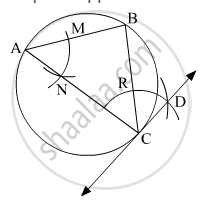Share

# Draw a Circle of Radius 3.6 Cm. Draw a Tangent to the Circle at Any Point on It Without Using the Centre. - Geometry

#### Question

Draw a circle of radius 3.6 cm. Draw a tangent to the circle at any point on it without using the centre.

#### Solution

Steps of Construction:
Step 1: Draw a circle with radius 3.6 cm. Mark any point C on it.
Step 2: Draw chord CB and an inscribed ∠CAB.
Step 3: With the centre A and any convenient radius draw an arc intersecting the sides of ∠BAC in points M and N.
Step 4: Using the same radius and centre C, draw an arc intersecting the chord CB at point R.
Step 5: Taking the radius equal to d(MN) and centre R, draw an arc intersecting the arc drawn in step 4. Suppose D be the point of intersection of these arcs. Draw line CD.Here, line CD is the required tangent to the circle.

Is there an error in this question or solution?